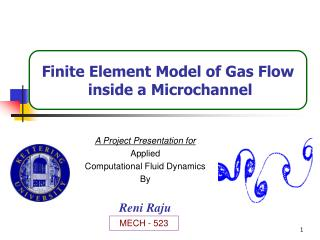DownloadDownload PresentationA Project Presentation for Applied Computational Fluid Dynamics By Reni Raju

# A Project Presentation for Applied Computational Fluid Dynamics By Reni Raju

Download Presentation## A Project Presentation for Applied Computational Fluid Dynamics By Reni Raju

- - - - - - - - - - - - - - - - - - - - - - - - - - - E N D - - - - - - - - - - - - - - - - - - - - - - - - - - -
##### Presentation Transcript

1. Finite Element Model of Gas Flow inside a Microchannel A Project Presentation for Applied Computational Fluid Dynamics By Reni Raju MECH - 523

2. Objectives • Develop a 2D Finite Element Model for gas flow inside a microchannel. • Develop FE formulation for N-S equations. • Implement Slip and temperature boundary conditions. • Compare Numerical and Experimental Data for Microchannel.

3. y +H/2 x -H/2 Microchannel Experimental data from Pong et. al (1994) Numerical data from Chen et al (1998)

4. Governing Equations Continuity Momentum Energy Equation of State

5. Normalized Equations Continuity Momentum Energy Equation of State

6. Kn=0.0001 0.001 0.01 0.1 1 10 100 Continuum Regime Transition Regime Slip Flow Regime Molecular Regime Flow Regimes Knudsen Number Gad-el-hak (1999)

7. Wall Conditions Wall Slip Maxwell (1879)

8. Thermal Boundary Condition Temperature Jump Von Smoluchowski (1898)

9. Finite Element Algorithm • Developed by the Computational Plasma Dynamics Laboratory at Kettering University (Roy, CMAME, v184, 87-98, 2000). • A Family of complex subroutines that can study macroscopic collisional plasmas. (Roy and Pandey, POP, v9, 4052-60, 2002). • Written in Fortran 77, use Cray-style Fortran pointers, and are designed for UNIX-type environment. • Two dimensional formulation (so far). • Implemented Sub-Grid Embedded (SGM) FE for Coarse-grid solution Stability,Accuracy and Tri-diagonal Efficiency (Roy and Baker, NHT-B, v33, 5-36, 1998). • Utilized to model Compressible flow through Electric Propulsion thrusters including Microchannels.

10. Numerical Details Problem Statement Weak Statement Discrete Approximation Nk is appropriate basis function; Chebyshev , Lagrange or Hermite interpolation polynomials complete to degree k.

11. FE Formulation Momentum Equation Variable Discretized Weak Statement

12. 1 7 4 3 6 8 9 1 2 5 Discretization FE Basis Cartesian Coordinate

13. Global/Local frame Element Jacobian Differential Element Diffusion Term Matrix Form

14. Solution Procedure FE Formulation Time Integration Form NR Iteration Convergence Criteria = 10-4 for all integrated quantities.

15. 1 7 4 3 6 8 9 1 2 5 Mesh • FE basis • 2D-Quadratic 9-node • 2D-Bilinear 4-node • Mesh • 1369 nodes • 324 elements 2 1 4 3 2 1 2

16. Code Format do i = 1,4 do j = 1,9 ii = loc_rho(i) jj = loc_v1(j) term = wt * rho * Nmat22(i) * DNmat33Dx(j) elemk_lin(ii,jj) = elemk_lin(ii,jj) + term jj = loc_v2(j) term = wt * epsi * rho * Nmat22(i) * DNmat33Dy(j) elemk_lin(ii,jj) = elemk_lin(ii,jj) + term enddo enddo do i = 1,4 do j = 1,4 ii = loc_rho(i) jj = loc_rho(j) term = wt * velx * Nmat22(i) * DNmat22Dx(j) elemk_lin(ii,jj) = elemk_lin(ii,jj) + term jj = loc_rho(j) term = wt * epsi * vely * Nmat22(i) * DNmat22Dy(j) elemk_lin(ii,jj) = elemk_lin(ii,jj) + term enddo enddo

17. Boundary conditions • At the Inlet • The Gas temperature Ti is specified as 314 K . • The y-component of the velocity v = 0 . • Inlet pressure, Pi is specified based on the corresponding Pressure ratio. • At the Outlet • The pressure at the outlet, P0 is 100.8 KPa. • On the Walls (No-slip) • For isothermal wall the wall temperature Tw is 314 K. • u and v velocity components = 0.

18. Microchannel Flow Contours Channel Aspect Ratio: 2500 Velocity Pressure Pressure ratios: 1.340, 1.680, 2.020, 2.361, 2.701

19. Slip/No-Slip Comparison Pressure Velocity Slip variation up to ~ +4% Slip variation up to ~ +8%

20. Pressure Distribution(Numerical-Experimental Comparison) Experimental validation within ~ 4 % Numerical validation within ~ 1.3 %

21. Velocity Profile(Numerical-Numerical Comparison) Numerical validation within ~ 2.5 %

22. y +H/2 x -H/2 Conclusion • For Microchannel- • Finite Element Model compares well with reported Numerical and Experimental results. • The slip conditions show higher flow rates.

23. Future Scope • Extend to the applicability of Slip-flow conditions to the Transition regime, ( 0.1 < Kn < 10 ). • Applicability to higher Knudsen number ranges (Nanoscale devices).

24. Acknowledgements • Dr. Subrata Roy. • Dr. Birendra Pandey. • Center of Nanotechnology, NASA Ames. • NSF/NPACI Supercomputer. • Electric Propulsion Laboratory, NASA Glenn Research Center.